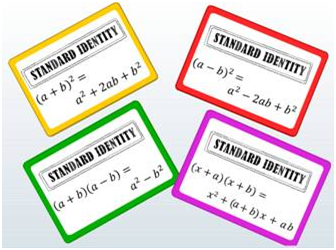Algebraic Identities
Identity: An equation is true for only certain values of the variables and need not be true for all values of the variables. If it is true for all values of the variables in it, then it is called an Identity. These identities are obtained on multiplying one binomial with another binomial and are known as Standard Identities. The four standard identities that we are going to learn in this lesson are:      •  (a + b)2 = a2 + 2ab + b2      •  (a - b)2 = a2 - 2ab + b2      •  (a + b)(a – b) = a2 – b2      •  (x + a)(x + b) = x2 + (a + b) x + ab#### Summary

Identity: An equation is true for only certain values of the variables and need not be true for all values of the variables. If it is true for all values of the variables in it, then it is called an Identity. These identities are obtained on multiplying one binomial with another binomial and are known as Standard Identities. The four standard identities that we are going to learn in this lesson are:      •  (a + b)2 = a2 + 2ab + b2      •  (a - b)2 = a2 - 2ab + b2      •  (a + b)(a – b) = a2 – b2      •  (x + a)(x + b) = x2 + (a + b) x + abPrevious
Next